AP Chemistry : Kinetics and Energy

Example Questions

Example Question #1 : Integrated Rate Laws

The rate constant for a second-order reaction is 0.15 M-1s-1.  If the initial concentration of the reactant is 0.30 M, how long does it take for the concentration to decrease to 0.15 M?

253 second

88.8 seconds

11.1 seconds

44.4 second

22.2 seconds

22.2 seconds

Explanation: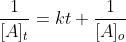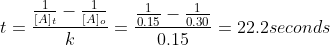Example Question #2 : Integrated Rate Laws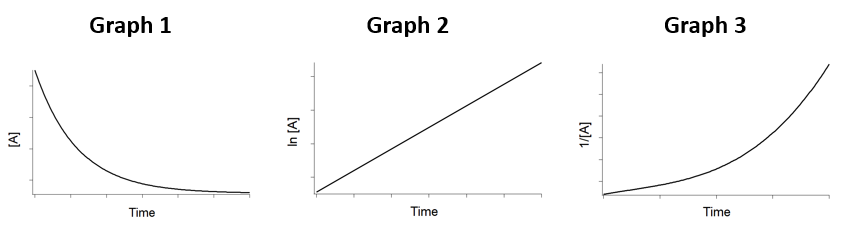Based on the figure above, what is the order of reaction?

Zero Order

Third Order

Squared Order

Second Order

First order

First order

Explanation:

For a first order reaction, the ln [A]t is linear with t.

Example Question #1 : Integrated Rate Laws

A compound decomposes by a first-order process.  If 25.0% of the compound decomposes in 60 minutes, the half-life of the compound is?

198 minutes

65 minutes

145 minutes

120 minutes

180 minutes

145 minutes

Explanation: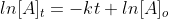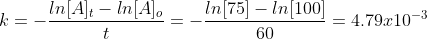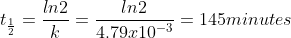Example Question #1 : Integrated Rate Laws

Cyclopentane is unstable and decomposes by a first order reaction.  The rate constant for this reaction is 9.5 s-1.  What is the half life of the reaction?

0.0729 second

0.0314 seconds

0.0582 seconds

0.132 seconds

0.0614 seconds

0.0729 second

Explanation: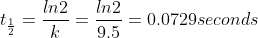Example Question #1 : Integrated Rate Laws

The half life of a first order reaction is 1.5 hours.  What is the rate constant of this reaction?

0.75

0.52

0.21

1.5

0.46

0.46

Explanation: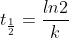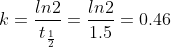Example Question #41 : Kinetics And Energy

Which of the following is true?

The rate-determining step of a reaction is the rate of the slowest elementary step of its mechanism

Since intermediate compounds can be formed, the chemical equations for the elementary reaction in a multistep mechanism do not always have to add to give the chemical equation of the overall process.

All of the above

In a reaction mechanism an intermediate is identical to an activated complex

If we know that a reaction is an elementary reaction, then we know its rate law.

All of the above

Explanation:

All of the above describe elementary reactions and how they give an overall mechanism.

Example Question #2 : Reaction Mechanisms

A possible mechanism for the overall reaction Br2 (g) + 2 NO (g)  ->  2 NOBr(g) is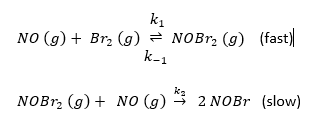The rate law for the formation of NOBr based on this mechanism is rate =           .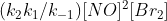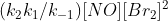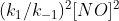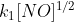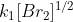Explanation:

Based on the slowest step the rate law would be:  Rate = k2 [NOBr2] [NO], but one cannot have a rate law in terms of an intermediate (NOBr2).

Because the first reaction is at equilibrium the rate in the forward direction is equal to that in the reverse, thus: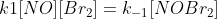and: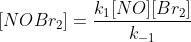Substitution yields: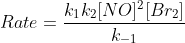Example Question #3 : Reaction Mechanisms

For the reaction NO2 (g) + CO (g) -> NO (g) + CO2 (g), the reaction was experimentally determined to be Rate = k[NO2]2.  If the reaction has the following mechanism, what is the rate limiting step, and why?

Step 1:  2 NO2  -> NO3 + NO                            (slow)

Step 2:  NO3 + CO -> NO2 + CO2                     (fast)

Step 1 is limiting because the reaction can not go faster than its slowest step.

Not enough information

Step 2 is limiting because the fast step determines how quickly the reaction can occur.

Step 1 is limiting because the NO2 is a reactant.

Step 2 is limiting because the NO3 intermediate has to be formed before the reaction can occur.

Step 1 is limiting because the reaction can not go faster than its slowest step.

Explanation:

The reaction can never go faster than its slowest step.

Example Question #651 : Ap Chemistry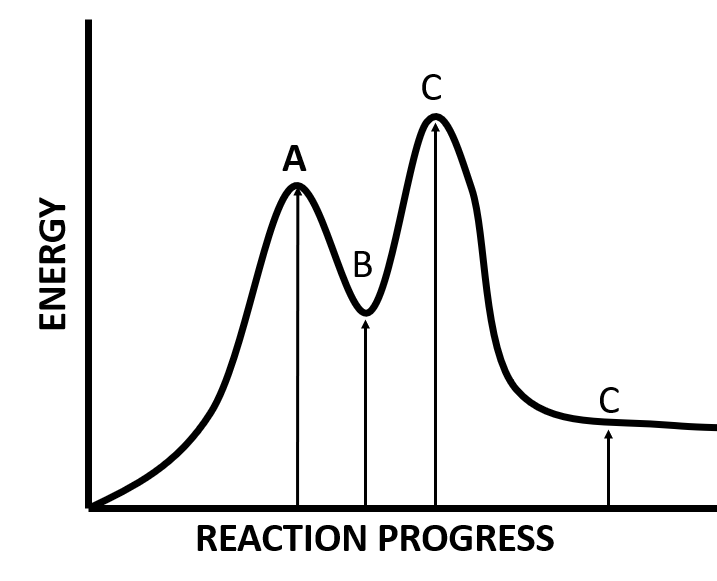Based on the figure above, what arrows corresponds to the activation energy of the rate limiting step and the energy of reaction?  Is the reaction endo- or exothermic?

Exothermic

Endothermic

Endothermic

Explanation:

Since the products are higher in energy than the reactions, the reaction is endothermic.

Example Question #652 : Ap Chemistry

Consider the following mechanism:

A + B ->  R +  C              (slow)

A + R  ->  C                   (fast)

There are no intermediates

C

A

B

R